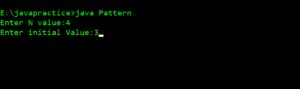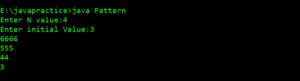# Print Right Triangle Number Pattern Type 3

## PRINT PATTERN  6*6*6*6 5*5*5 4*4 3

For any input number N Print the following code – For below code N=4 and Initial value is 3

```6*6*6*6
5*5*5
4*4
3
```

### PREREQUISITE:

Basic knowledge in Java programming, usage of loops.

### ALGORITHM:

1. Take input from user i.e number of lines required (N value) and initial value ‘a’ and copy n value to ‘t’.
2. Take two loops one for each line (say ‘i’) and other for each digit in a particular line (say ‘j’). i starts from (n+a)-1 and j starts from 1.
3. Here ‘i’ loop is used to access each line from 1 to n and ‘j’ loop is used to print values in each line. j loop is executed until it reaches i value.
4. Print ‘i’ value until the j loop reaches t value and decrement t and .go to next line.
5. Repeat loop until it reaches a value.

### CODE IN JAVA:

`import java.lang.*;import java.io.*;class Main{    public static void main (String[]args) throws IOException    {        BufferedReader br =new BufferedReader (new InputStreamReader (System.in));        int n, i, j, a, t;        System.out.print ("Enter N value:");        n = Integer.parseInt (br.readLine ());        t = n;        System.out.print ("Enter initial Value:");        a = Integer.parseInt (br.readLine ());            for (i = (n + a) - 1; i >= a; i--)        {	        for (j = 1; j <= t; j++)	        {	            System.out.print (i);	        }	        System.out.println ();	        t--;        }    }}`

### TAKING INPUT:### DISPLAYING OUTPUT: